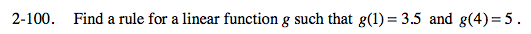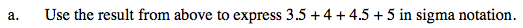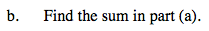### Home > PC > Chapter 2 > Lesson 2.3.3 > Problem2-100

2-100.Find the slope of the line going through g(1) and g(4).

$\text{slope }=\frac{5-3.5}{4-1}=\frac{1.5}{3}=0.5$

Substitute the point and the slope in the point-slope form to find the equation.

(g(x) − 5) = 0.5(x − 4)
g(x) = 0.5x − 2 + 5
g(x) = 0.5x + 3

Write the Sigma notation.

$\displaystyle\sum\limits_{k=1}^40.5k+3$